Documentation

# parallelplot

Create parallel coordinates plot

## Syntax

``p = parallelplot(tbl)``
``p = parallelplot(tbl,'CoordinateVariables',coordvars)``
``p = parallelplot(___,'GroupVariable',grpvar)``
``p = parallelplot(data)``
``p = parallelplot(data,'CoordinateData',coorddata)``
``p = parallelplot(___,'GroupData',grpdata)``
``p = parallelplot(___,Name,Value)``
``p = parallelplot(parent,___)``

## Description

example

````p = parallelplot(tbl)` creates a parallel coordinates plot from the table `tbl` and returns a `ParallelCoordinatesPlot` object. Each line in the plot represents a row in the table, and each coordinate variable in the plot corresponds to a column in the table. The software plots all table columns by default.Use `p` to modify the object after you create it. For a list of properties, see ParallelCoordinatesPlot Properties.```

example

````p = parallelplot(tbl,'CoordinateVariables',coordvars)` creates a parallel coordinates plot from the `coordvars` variables in the table `tbl`.```

example

````p = parallelplot(___,'GroupVariable',grpvar)` uses the table variable specified by `grpvar` to group the lines in the plot. Specify this option after any of the input argument combinations in the previous syntaxes.```

example

````p = parallelplot(data)` creates a parallel coordinates plot from the numeric matrix `data`.```

example

````p = parallelplot(data,'CoordinateData',coorddata)` creates a parallel coordinates plot from the `coorddata` columns in the matrix `data`.```

example

````p = parallelplot(___,'GroupData',grpdata)` uses the data in `grpdata` to group the lines in the plot. Specify this option after any of the previous input argument combinations for numeric matrix data.```

example

````p = parallelplot(___,Name,Value)` specifies additional options using one or more name-value pair arguments. For example, you can specify the data normalization method for coordinates with numeric values. For a list of properties, see ParallelCoordinatesPlot Properties.```
````p = parallelplot(parent,___)` creates the parallel coordinates plot in the figure, panel, or tab specified by `parent`.```

## Examples

collapse all

Create a parallel coordinates plot from a table of medical patient data.

Load the `patients` data set, and create a table from a subset of the variables loaded into the workspace. Create a parallel coordinates plot using the table. The lines in the plot correspond to individual patients. Use the plot to observe trends in the data. For example, the plot indicates that smokers tend to have higher blood pressure values (both diastolic and systolic).

```load patients tbl = table(Diastolic,Smoker,Systolic); p = parallelplot(tbl)``````p = ParallelCoordinatesPlot with properties: SourceTable: [100x3 table] CoordinateVariables: {'Diastolic' 'Smoker' 'Systolic'} GroupVariable: '' Show all properties ```

By default, the software randomly jitters plot lines so that they are unlikely to overlap perfectly along coordinate rulers. This jittering is particularly helpful for visualizing categorical data because it enables you to distinguish between plot lines more easily. For example, observe the plot lines along the `Smoker` coordinate ruler; the plot lines are not flush with either the `true` or `false` tick marks.

To disable the default jittering, set the `Jitter` property to `0`.

`p.Jitter = 0;`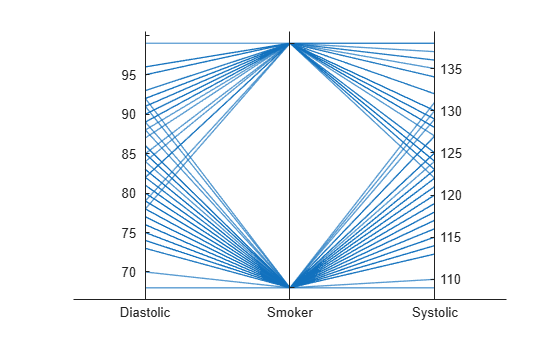Create a parallel coordinates plot from a table of tsunami data. Specify the table variables to display and their order, and group the lines in the plot according to one of the variables.

Read the tsunami data into the workspace as a table.

`tsunamis = readtable('tsunamis.xlsx');`

Create a parallel coordinates plot using a subset of the variables in the table. First, increase the figure window size to prevent overcrowding in the plot. Then, to specify the variables and their order, use the `'CoordinateVariables'` name-value pair argument. To group occurrences according to their validity, set the `'GroupVariable'` name-value pair argument to `'Validity'`. The lines in the plot correspond to individual tsunami occurrences. The plot indicates that most of the occurrences in the data set that have a `Validity` value are considered definite tsunamis.

```figure('Units','normalized','Position',[0.3 0.3 0.45 0.4]) coordvars = {'Year','Validity','Cause','Country'}; p = parallelplot(tsunamis,'CoordinateVariables',coordvars,'GroupVariable','Validity');```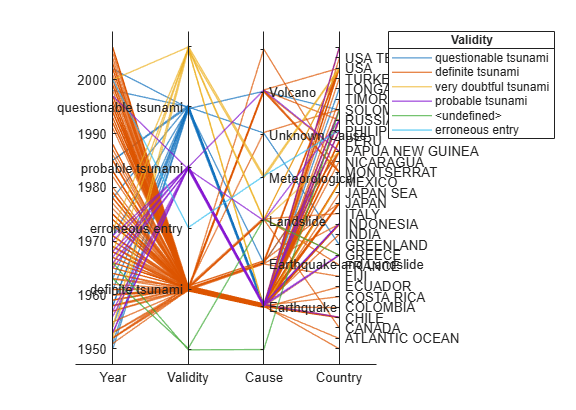Create a parallel coordinates plot from a matrix containing medical patient data. Bin the values in one of the columns in the matrix, and group the lines in the plot using the binned values.

Load the `patients` data set, and create a matrix from the `Age`, `Height`, and `Weight` values. Create a parallel coordinates plot using the matrix data. Label the coordinate variables in the plot. The lines in the plot correspond to individual patients.

```load patients X = [Age Height Weight]; p = parallelplot(X)```
```p = ParallelCoordinatesPlot with properties: Data: [100x3 double] CoordinateData: [1 2 3] GroupData: [] Show all properties ```
`p.CoordinateTickLabels = {'Age (years)','Height (inches)','Weight (pounds)'};`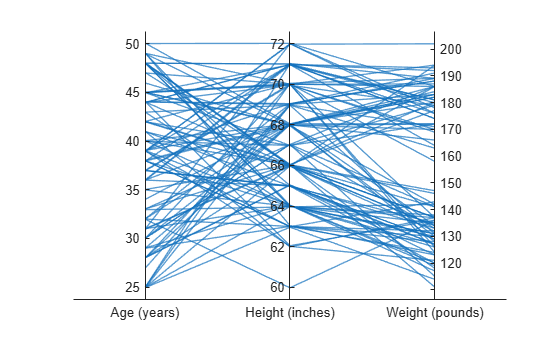Create a new categorical variable that groups each patient into one of three categories: `short`, `average`, or `tall`. Set the bin edges such that they include the minimum and maximum `Height` values.

`min(Height)`
```ans = 60 ```
`max(Height)`
```ans = 72 ```
```binEdges = [60 64 68 72]; bins = {'short','average','tall'}; groupHeight = discretize(Height,binEdges,'categorical',bins);```

Now use the `groupHeight` values to group the lines in the parallel coordinates plot. The plot indicates that `short` patients tend to weigh less than `tall` patients.

`p.GroupData = groupHeight;`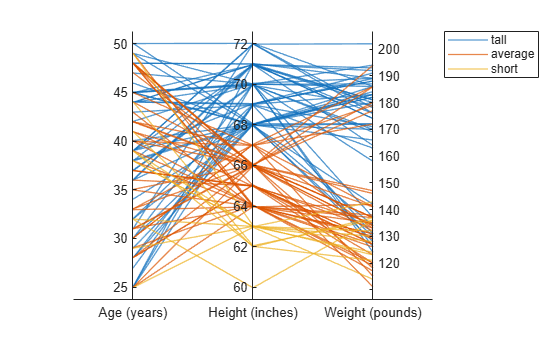Create parallel coordinates plots from a matrix containing medical patient data. For each plot, specify the columns of the matrix to display, and group the lines in the plot according to a separate variable.

Load the `patients` data set, and create a matrix from some of the variables loaded into the workspace.

```load patients X = [Age Height Weight];```

Create a parallel coordinates plot using a subset of the columns in the matrix `X`. To specify the columns and their order, use the `'CoordinateData'` name-value pair argument. Group patients according to their smoker status by passing the `Smoker` values to the `'GroupData'` name-value pair argument. The lines in the plot correspond to individual patients. The plot indicates that no clear relationship exists between smoker status and either age or weight.

```coorddata = [1 3]; p = parallelplot(X,'CoordinateData',coorddata,'GroupData',Smoker)```
```p = ParallelCoordinatesPlot with properties: Data: [100x3 double] CoordinateData: [1 3] GroupData: [100x1 logical] Show all properties ```
`p.CoordinateTickLabels = {'Age','Weight'};`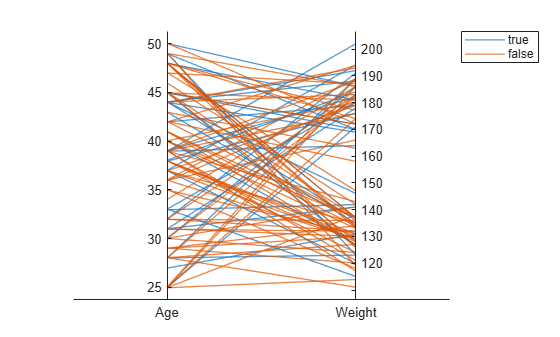Create another parallel coordinates plot using a different subset of the columns in `X`. Group the patients according to their gender. The plot indicates that the men are taller and weigh more than the women.

```coorddata2 = [2 3]; p2 = parallelplot(X,'CoordinateData',coorddata2,'GroupData',Gender)```
```p2 = ParallelCoordinatesPlot with properties: Data: [100x3 double] CoordinateData: [2 3] GroupData: {100x1 cell} Show all properties ```
`p2.CoordinateTickLabels = {'Height','Weight'};`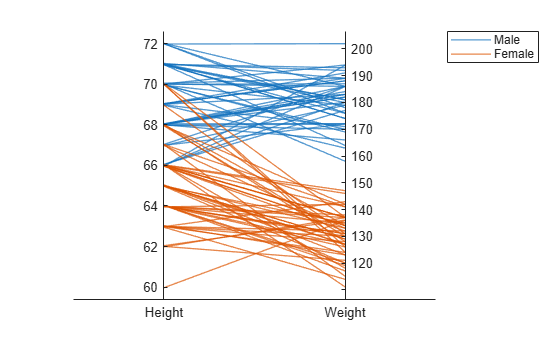Create a parallel coordinates plot from a table of power outage data. Change the normalization method for the numeric coordinate variables.

Read the power outage data into the workspace as a table. Display the first few rows of the table.

```outages = readtable('outages.csv'); head(outages)```
```ans=8×6 table Region OutageTime Loss Customers RestorationTime Cause _____________ ________________ ______ __________ ________________ ___________________ {'SouthWest'} 2002-02-01 12:18 458.98 1.8202e+06 2002-02-07 16:50 {'winter storm' } {'SouthEast'} 2003-01-23 00:49 530.14 2.1204e+05 NaT {'winter storm' } {'SouthEast'} 2003-02-07 21:15 289.4 1.4294e+05 2003-02-17 08:14 {'winter storm' } {'West' } 2004-04-06 05:44 434.81 3.4037e+05 2004-04-06 06:10 {'equipment fault'} {'MidWest' } 2002-03-16 06:18 186.44 2.1275e+05 2002-03-18 23:23 {'severe storm' } {'West' } 2003-06-18 02:49 0 0 2003-06-18 10:54 {'attack' } {'West' } 2004-06-20 14:39 231.29 NaN 2004-06-20 19:16 {'equipment fault'} {'West' } 2002-06-06 19:28 311.86 NaN 2002-06-07 00:51 {'equipment fault'} ```

Create a new variable called `OutageDuration` that indicates how long each power outage lasted. Convert `OutageDuration` to the number of days each power outage lasted. Add the new variable to the `outages` table, and call it `OutageDays`.

```OutageDuration = outages.RestorationTime - outages.OutageTime; outages.OutageDays = days(OutageDuration);```

Create a parallel coordinates plot using the `Loss`, `Customers`, and `OutageDays` variables. Because the coordinate variables are numeric, display the values in the plot as z-scores, without any jittering, using the `'DataNormalization'` and `'Jitter'` name-value pair arguments.

```coordvars = {'Loss','Customers','OutageDays'}; p = parallelplot(outages,'CoordinateVariables',coordvars,'DataNormalization','zscore','Jitter',0);```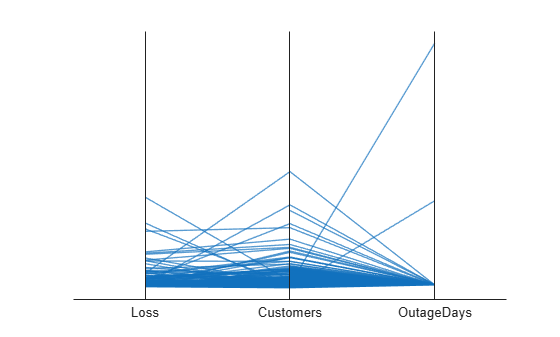The `OutageDays` variable contains one value that is more than 30 standard deviations away from the mean `OutageDays` value and another value that is more than 10 standard deviations away from the mean. Hover over the values in the plot to display data tips. Each data tip indicates the row in the table corresponding to the line in the plot.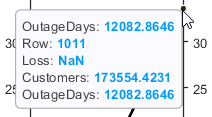Find the rows in the `outages` table that have the identified extreme `OutageDays` values. Notice that the `RestorationTime` values for these two power outages are suspicious.

`outliers = outages([1011 269],:)`
```outliers=2×7 table Region OutageTime Loss Customers RestorationTime Cause OutageDays _____________ ________________ ______ __________ ________________ ____________________ __________ {'NorthEast'} 2009-08-20 02:46 NaN 1.7355e+05 2042-09-18 23:31 {'severe storm' } 12083 {'MidWest' } 2008-02-07 06:18 2378.7 0 2019-08-14 16:16 {'energy emergency'} 4206.4 ```

Create a parallel coordinates plot. Reorder the categories of one of the coordinate variables.

Read data on power outages into the workspace as a table.

`outages = readtable('outages.csv');`

Create a parallel coordinates plot using a subset of the columns in the table. Group the lines in the plot according to the event that caused the power outage.

```coordvars = [1 3 4 6]; p = parallelplot(outages,'CoordinateVariables',coordvars,'GroupVariable','Cause');```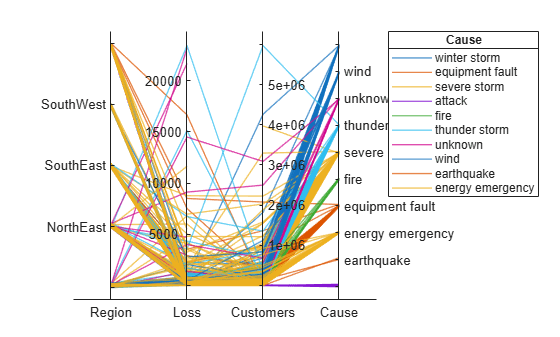Change the order of the events in `Cause` by updating the source table. First, convert `Cause` to a `categorical` variable, specify the new order of the events, and use the `reordercats` function to create a new variable called `orderCause`. Then, replace the original `Cause` variable with the new `orderCause` variable in the source table of the plot.

```categoricalCause = categorical(p.SourceTable.Cause); newOrder = {'attack','earthquake','energy emergency','equipment fault', ... 'fire','severe storm','thunder storm','wind','winter storm','unknown'}; orderCause = reordercats(categoricalCause,newOrder); p.SourceTable.Cause = orderCause;```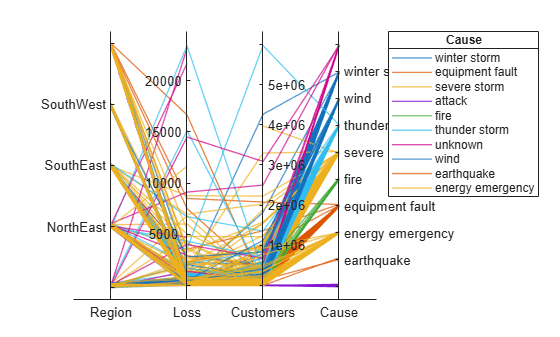Because the `Cause` variable contains more than seven categories, some of the groups have the same color in the plot. Assign distinct colors to every group by changing the `Color` property of `p`.

`p.Color = parula(10);`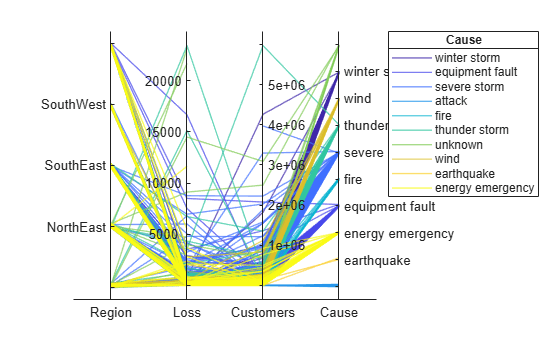## Input Arguments

collapse all

Source table, specified as a table.

You can create a table from workspace variables using the `table` function, or you can import data as a table using the `readtable` function.

The `SourceTable` property of the `ParallelCoordinatesPlot` object stores the source table.

Table variables to display as coordinates, specified in one of these forms:

• Numeric vector — Indicating the indices of the table variables. For example, `parallelplot(tbl,'CoordinateVariables',[1 5:7])` selects the first, fifth, sixth, and seventh variables in the table to display as coordinates.

• String array or cell array of character vectors — Indicating the names of the table variables. For example, `parallelplot(tbl,'CoordinateVariables',{'Age','Weight','Height'})` selects the variables named `'Age'`, `'Weight'`, and `'Height'` to display as coordinates.

• Logical vector — Containing `true` elements for the selected table variables.

The `CoordinateVariables` property of the `ParallelCoordinatesPlot` object stores the `coordvars` value. The `CoordinateTickLabels` property stores the selected variable names.

Table variable for grouping data, specified in one of these forms:

• Character vector or string scalar — Indicating one of the table variable names

• Numeric scalar — Indicating the table variable index

• Logical vector — Containing one `true` element for the table variable

The values associated with your table variable must form a numeric vector, logical vector, categorical array, string array, or cell array of character vectors.

`grpvar` splits the rows in `tbl` into unique groups. By default, the software colors the associated plot lines according to their group value. Plot lines corresponding to the same group have the same color. However, `parallelplot` assigns a maximum of seven unique group colors. When the total number of groups exceeds the number of specified colors, `parallelplot` cycles through the specified colors.

In the legend, `parallelplot` displays the group names in order of their first appearance in the `GroupData` property of `ParallelCoordinatesPlot`.

Example: `'Smoker'`

Example: `3`

Input data, specified as a numeric matrix.

The `Data` property of the `ParallelCoordinatesPlot` object stores the `data` values.

Matrix columns to display as coordinates, specified in one of these forms:

• Numeric vector — Indicating the columns of the input data matrix. For example, `parallelplot(data,'CoordinateData',[1 5:7])` selects the first, fifth, sixth, and seventh columns in `data` to display as coordinates.

• Logical vector — Containing `true` elements for the selected columns of the input data matrix.

The `CoordinateData` property of the `ParallelCoordinatesPlot` object stores the `coorddata` value.

Values for grouping matrix data, specified as a numeric vector, logical vector, categorical array, string array, or cell array of character vectors.

`grpdata` splits the rows in `data` into unique groups. By default, the software colors the associated plot lines according to their group value. Plot lines corresponding to the same group have the same color. However, `parallelplot` assigns a maximum of seven unique group colors. When the total number of groups exceeds the number of specified colors, `parallelplot` cycles through the specified colors.

In the legend, `parallelplot` displays the group names in order of their first appearance in the `GroupData` property of `ParallelCoordinatesPlot`.

Example: `[1 2 1 3 2 1 3 3 2 3]`

Example: `categorical({'blue','red','yellow','blue','yellow','red','red','yellow','blue','red'})`

Parent container, specified as a `Figure`, `Panel`, `Tab`, or `TiledChartLayout` object.

### Name-Value Pair Arguments

Specify optional comma-separated pairs of `Name,Value` arguments. `Name` is the argument name and `Value` is the corresponding value. `Name` must appear inside quotes. You can specify several name and value pair arguments in any order as `Name1,Value1,...,NameN,ValueN`.

Example: `parallelplot(data,'GroupData',grpdata,'DataNormalization','zscore','Jitter',0)` specifies to group the numeric data in `data` by using `grpdata` and to display the data as z-scores, without any jittering.

Plot title, specified as a character vector, string array, cell array of character vectors, or categorical array. By default, the plot has no title.

To create a multiline title, specify a string array or cell array of character vectors. Each element in the array corresponds to a line of text.

If you specify the title as a categorical array, MATLAB® uses the values in the array, not the categories.

Example: `p = parallelplot(__,'Title','My Title Text')`

Example: `p.Title = 'My Title Text'`

Example: `p.Title = {'My','Title'}`

Normalization method for coordinates with numeric values, specified as one of the following options.

MethodDescription
`'range'`Display raw data along coordinate rulers that have independent minimum and maximum limits
`'none'`Display raw data along coordinate rulers that have the same minimum and maximum limits
`'zscore'`Display z-scores (with a mean of 0 and a standard deviation of 1) along each coordinate ruler
`'scale'`Display values scaled by standard deviation along each coordinate ruler
`'center'`Display data centered to have a mean of 0 along each coordinate ruler
`'norm'`Display 2-norm values along each coordinate ruler

For more information about these methods, see `normalize`.

For a coordinate variable that is a logical vector, datetime array, duration array, categorical array, string array, or cell array of character vectors, `parallelplot` evenly distributes the unique possible values along the coordinate ruler, regardless of the normalization method.

Example: `p = parallelplot(__,'DataNormalization','none')`

Example: `p.DataNormalization = 'zscore'`

Data displacement distance along the coordinate rulers, specified as a numeric scalar in the interval [0,1]. The `Jitter` value determines the maximum distance to displace plot lines from their true value along the coordinate rulers, where the displacement is a uniform random amount. If you set the `Jitter` property to `1`, then adjacent jitter regions just touch. Set the `Jitter` property to `0` to display the true data values.

Some amount of jitter is particularly helpful for visualizing categorical data because the jittering enables you to distinguish between plot lines more easily. However, the `Jitter` value affects all coordinate variables, including numeric variables.

Example: `p = parallelplot(__,'Jitter',0.5)`

Example: `p.Jitter = 0.2`

Group color, specified in one of these forms:

• Character vector designating a color name, short name, or hexadecimal color code. A hexadecimal color code starts with a hash symbol (`#`) and is followed by three or six hexadecimal digits, which can range from `0` to `F`. The values are not case sensitive. Thus, the color codes `'#FF8800'`, `'#ff8800'`, `'#F80'`, and `'#f80'` are equivalent.

• String array or cell array of character vectors designating one or more color names, short names, or hexadecimal color codes.

• Three-column matrix of RGB values in the range [0,1]. The three columns represent the R value, G value, and B value.

Choose among these predefined colors, their equivalent RGB triplets, and their hexadecimal color codes.

Color NameShort NameRGB TripletHexadecimal Color CodeAppearance
`'red'``'r'``[1 0 0]``'#FF0000'``'green'``'g'``[0 1 0]``'#00FF00'``'blue'``'b'``[0 0 1]``'#0000FF'``'cyan'` `'c'``[0 1 1]``'#00FFFF'``'magenta'``'m'``[1 0 1]``'#FF00FF'``'yellow'``'y'``[1 1 0]``'#FFFF00'``'black'``'k'``[0 0 0]``'#000000'``'white'``'w'``[1 1 1]``'#FFFFFF'`Here are the RGB triplets and hexadecimal color codes for the default colors MATLAB uses in many types of plots.

`[0 0.4470 0.7410]``'#0072BD'``[0.8500 0.3250 0.0980]``'#D95319'``[0.9290 0.6940 0.1250]``'#EDB120'``[0.4940 0.1840 0.5560]``'#7E2F8E'``[0.4660 0.6740 0.1880]``'#77AC30'``[0.3010 0.7450 0.9330]``'#4DBEEE'``[0.6350 0.0780 0.1840]``'#A2142F'`By default, `parallelplot` assigns a maximum of seven unique group colors. When the total number of groups exceeds the number of specified colors, `parallelplot` cycles through the specified colors.

Example: `p = parallelplot(__,'Color',{'blue','black','green'})`

Example: `p.Color = [0 0 1; 0 0.5 0.5; 0.5 0.5 0.5]`

Example: `p.Color = {'#EDB120','#77AC30','#7E2F8E'}`

## Tips

• To interactively explore the data in your `ParallelCoordinatesPlot` object, use these options (some are not available in the Live Editor):

• Zoom — Use the scroll wheel to zoom.

• Pan — Click and drag the parallel coordinates plot to pan.

• Data tips — Hover over the parallel coordinates plot to display a data tip. The software highlights the corresponding line in the plot. For an example, see Change Data Normalization in Plot.

• Rearrange coordinates — Click and drag a coordinate tick label horizontally to move the corresponding coordinate ruler to a different position. For an example, see Explore Table Data Using Parallel Coordinates Plot.